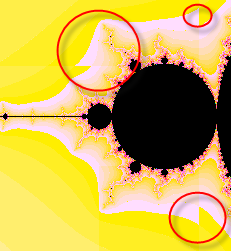## Friday, April 28, 2006

### Strange floating point behavior on Xeon

One of the first applications I wrote using the Digipede Framework API was a distributed Mandelbrot app. It's almost a cliche application for a grid computing system--still, it looks pretty and calculates a heck of a lot faster on many machines than on one.

Recently, I was tweaking our calculation code to get better colors out of it. After tweaking it, I noticed something very strange happening: different machines were calculating different colors for the same pixels! Here's a screencap that shows how it manifested itself:Because different machines calculate different portions of the picture, and those portions may end up right next to each other, it's pretty obvious. The rough edges give you an idea that things just weren't right--I've circled some of them.

At some point, I realized that there was one machine on my testbed in particular that was returning different values than the others. I also noticed that the gradients on the bitmaps from that machine lined up perfectly with the gradients on the bitmaps from the other machines--the colors were just different. Furthermore, the colors were always 1 color off (the colors in my Mandelbrot are stored in an array).As it turns out, that machine has dual Xeon processors in it, and it's the only one on our network that does. It was pretty clear that the Xeons were causing a problem--and today I decided to pin it town.

To calculate a Mandelbrot set you do a repetitive calculation using complex math. Basically, you start with a complex number z, and you iteratively perform the calculation z = (z * z) + c. You count the number of times you can do that before z is too large to calculate. You then choose your color based on the number of iterations.

I added some code to my calculation object to spit out the results from the calculations. On most machines, it looked like this:

after 0: z = -1.78571428571429 + -1.19047619047619i
after 1: z = -0.0141723356009069 + 3.06122448979592i
after 2: z = -11.1566088075442 + -1.2772455921144i
after 3: z = 121.052849496283 + 27.3089826542847i
after 4: z = 13906.2261232719 + 6610.46985810096i
after 5: z = 149684811.460994 + 183853376.065174i
after 6: z = -1.1396521108449E+16 + 5.50401158655656E+16i
after 7: z = -2.89953366111956E+33 + -1.25453168454679E+33i
after 8: z = 6.83344570443359E+66 + 7.27511369656889E+66i
after 9: z = -6.23129910256229E+132 + 9.94281888781693E+133i
after 10: z = -9.84713565508731E+267 + -1.23913356825186E+267i
after 11: z = Infinity + Infinityi
But on the Xeon machine, it looked like this:
after 0: z = -1.78571428571429 + -1.19047619047619i
after 1: z = -0.0141723356009069 + 3.06122448979592i
after 2: z = -11.1566088075442 + -1.2772455921144i
after 3: z = 121.052849496283 + 27.3089826542847i
after 4: z = 13906.2261232719 + 6610.46985810096i
after 5: z = 149684811.460994 + 183853376.065174i
after 6: z = -1.1396521108449E+16 + 5.50401158655656E+16i
after 7: z = -2.89953366111956E+33 + -1.25453168454679E+33i
after 8: z = 6.83344570443359E+66 + 7.27511369656889E+66i
after 9: z = -6.23129910256229E+132 + 9.94281888781693E+133i
after 10: z = -9.84713565508731E+267 + -1.23913356825186E+267i
after 11: z = NaN + Infinityi
after 12: z = NaN + NaNi
See the difference? After 10 iterations, the calculations were identical. But on the 11th, the first goes to Infinity. The second, though, goes to NaN!

According to the .NET 2.0 documentation on NaN, "This constant is returned when the result of an operation is undefined." And the documentation for Double.PositiveInfinity says "This constant is returned when the result of an operation is greater than MaxValue." Double.NegativeInfinity is, of course, "This constant is returned when the result of an operation is less than MinValue."

MaxValue, by the way, is 1.79769313486232e308. So there's no doubt that the result of squaring z was going to overflow. But I don't see anything to indicate that the result should be NaN--and all of my other machines agree: it should be Infinity.

So is this a problem with the Xeon? The FPU? The .NET 2.0 libraries on my x64 machine? It's a 32-bit app, so I don't see how that would be.

I was able to work around this in code, but it's not the kind of thing developers should have to worry about (see Kim's post on how developers rely on the systems beneath their code).

Still - I'm glad this was only an application drawing pretty pictures. I'd hate to see the calculations on the trajectory of our next Mars probe to go awry based on something like this.

Has anyone else had any discrepancies using .NET 2.0 on the Xeon?

Technorati tags: , , ,